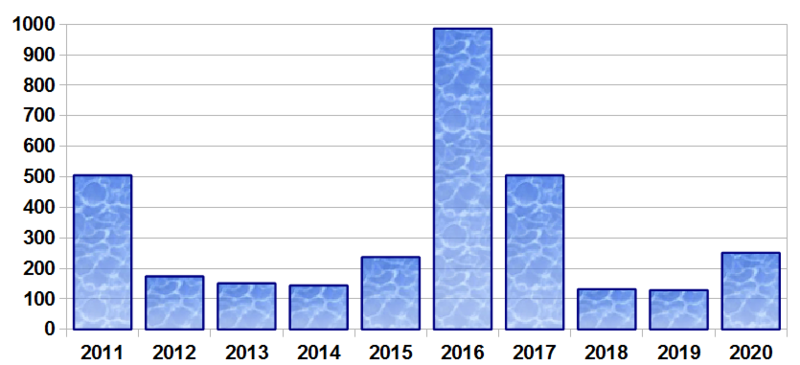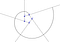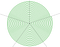# Happy 2000+10+10!

You, who are part of the part of humanity that uses the Gregorian calendar, you have just passed the year 2020! It is therefore legitimate that I wish you a very happy new year 2020!

Do I still have late work? Yes of course. Will I post them on time? It would be a good resolution to take. But there is a resolution that I will not put aside anytime soon, answering the question that everyone is asking in this year 2020: will this new year be interesting?

The methodology is always the same. A year is interesting if and only if it has many occurrences in the OEIS, the online encyclopedia of whole sequences. The years 2018 and 2019 were fairly arbitrary, with only a hundred interesting properties. Yet 2020 will be …

# Not bad.No fabulous, but not bad.Number of properties listed by the OEIS for each year.

With 251 properties, 2020 is the fourth most interesting year of the decade. Its decomposition into product of prime factors is 2020 = 2 × 2 × 5 × 101, which prevents it from benefiting from the properties inherent in the first years, but thanks to its only digits 0 and 2, it recovers properties of the numbers written in base 3 (and there are many).

Let’s take a closer look at some of the properties that make this 2020 number unique.

## 2020 is a curious autobiographical number [A046043]

A number is self-describing if, as the name suggests, it describes itself. This is, for example, the case of the number 10153331, which has 1 time the number 0, 1 time the number 5, 3 times the number 3 and 3 times the number 1. Note that 10153331 is the smallest prime number to check this property. There is only a finite number of self-describing numbers, ranging from 22 to 9998979595959595848484848484848476737373737373736262626262625151515110 (you can verify that this number has 9 times the number 9, 9 times the number 7, etc.)

There are autobiographical numbers. The number 10213223 is autobiographical, since it describes itself in order: it has 1 time the number 0, 2 times the number 1, 3 times the number 2 and 2 times the number 3. The described figures ( in italic) are here in ascending order, which makes the number 10213223 the smallest integer (after 22) to check this property. Such a number cannot have more than 20 digits, the largest of which is 101112213141516171819.

The number 2020 is a curious autobiographical number, but in a slightly different way, since the figures described (in italic) are implied. In the number 2020, there are 2 times the number 0, 0 times the number 1, 2 times the number 2 and 0 times the number 3. There are only 7 curious autobiographical numbers, 2020 is the second (the first being 1210).

## A spiral with 5 centers after 20 turns has a length of 2020π [A202803]

Let’s build a 5-center spiral. For this, we start from a regular pentagon ABCDE of side 1 from which we construct 5 half-lines [BA), [CB); [DC), [ED) and [AE). We then build between the half-lines [BA) and [CB) an arc centered in B passing through A, then we extend it between the following half-lines by centering it on the vertex following C, and so on. After 5 arcs, the constructed spiral makes a turn and one can calculate its length: the first constructed arc has indeed a radius of 1, the following of 2 and so on. Each arc describing an angle of 2π / 5, we deduce that the length of the spiral after a turn is 1 × 2π / 5 + 2 × 2π / 5 + 3 × 2π / 5 + 4 × 2π / 5 + 5 × 2π / 5 = (1 + 2 + 3 + 4 + 5) × 2π / 5 = 6π.Spiral step 1.

Generally, if the spiral do n turns, its length will be [1 + 2 + 3 + … + 5n] × 2π / 5 = [5n × (5n + 1) / 2] × 2π / 5 = n × (5n + 1) π. For 20 turn, this gives a length of 2020 π.Spiral with 5 centers and 20 turns. Its length is 2020π.

In each portion of the plane delimited by the half-lines, the arcs of circles are concentric.

## The number 12 has 2020 over cubic scores [A246584]

How many ways are there to write the number 4 as a decreasing sum of non-zero integers? detail

4 = 1+1+1+1
= 2+1+1
= 2+2
= 3+1
= 4

So there are 5 ways to write 4 as a sum. So we say that 4 has 5 partitions. These partitions were particularly studied by the mathematician of Indian origin Ramanujan who drew many properties from them. One of them, the “Ramanujan congruence”, indicates that for a number out of 5, the partitions are divisible by 5 (more precisely, if we note p (n) the number of partitions of n, then p (5k +4) = 0 [mod 5]). The study of partitions has also led Ramanujan to study his generative series, given by the following pretty formula:

And if, for some obscure reason, we consider the following decompositions:

In this case, the number of partitions is much greater, since it amounts to 26:

We then say that 4 has 26 “over cubic partitions”, which is a notion of number theory which is difficult at first to understand the motivations that led to its existence. In reality, what led to the introduction of these partitions is their generative series, which generalizes that of the partitions, and which makes it possible to study the Ramanujan congruences from a new angle.

But also…

• 2020 is the concatenation of a pair of identical numbers, just like 1515 or 4242 (A020338).
• The sum of the digits of 2020 is equal to its number of digits (A061384).
• the number 2020³ has only even digits (which is oddly rather rare) (A052004).
• 2020 = 17² + 19² + 23² + 29², which is the sum of four consecutive prime numbers (A133524).

Read,vwrite, repeat...

## More from Mao Tss

Read,vwrite, repeat...